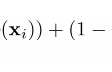# What’s Recursion?

Recursion is a powerful concept and it is said to occur when an entity calls itself or things define in terms of themselves. Recursion is found in diverse subjects: arts, computers, mathematics, logic, toys, games and linguistic.

Drawings of M. C. Escher are known to portray recursion. Check out this painting: a painting drawing itself in the painting.

There are numerous examples of Droste effect where a picture is having the same pictures inside in a stack form, calling itself one after another: images of doors and windows calling themselves.

Recursion occurs in food, too. Sourdough, Greek yogurt and curd are prepared by using the remains of themselves. That way, logically, reproduction is recursion only. A human produces a human/s. A lion produces a lion/s. A plant produces plant/s. A virus produces virus/es.

Recursive is an adjective of recursion and it has originated from the Latin verb “recurrere” meaning “to run back.” There is recursive humor, recursive grammar, recursive definition (in mathematics and computer science) and there is Recursion, a novel by Blake Crouc.

Professor Eric Grimson of MIT has defined recursion in two ways-

1- Algorithmically: a way to design solutions to problems by divide-and-conquer or decrease-and-conquer i.e. reducing the problem into a smaller versions of the same problems.

2- Semantically: a programming technique where a function calls itself. And there has to be a way to exit else it’s going to be an endless loop or an infinite recursion. So the program must have:

a) one or more base cases that should be easy to solve.

b) solve the same problem with some other input having the goal to simplify the input of larger problem/s.

PS: It’s better to have a thorough understanding of linked lists, queues and stacks before you take a plunge in recursion.

## Why programmers use recursion?

a) It’s one of the most powerful data-structure.

b) It comes handy when one needs to solve complex problems.

c) Saves time.

d) Too little coding lines. So the code looks neat.

Let’s learn it by resolving a few problems in python.

# Recursive Programming in Python

## Problem 1- Factorial:

The factorial of a positive integer n is denoted by n!. Factorial is the product of all positive integers less than or equal to n. It’s written as —

n! = n * (n-1)! if n > 1 and f(1) = 1

e.g.

5! = 5 * 4 * 3 * 2 * 1 = 120

We have defined a function fact(n) and it calls fact(n-1) the recursive case till n==1 i.e the basecase. It returns 1 when n ==1. That’s the exit condition else it will be an infinite recursion.

## Problem 2- Fibonacci Series

Factorial has one basecase but Fibonacci Series has two basecases. The Fibonacci Series is includes these attributes:

1. The first element is 0. (basecase)
2. The second element is 1.(basecase)
3. The nth element is the sum of the (n-1)th and (n-2)th elements. (recursive case)

Henceforth, the third number in the sequence is the sum of the first and the second number: 0+1=1. And the 4th number is sum of the second and the third number: 1+1=2 and so on and so forth.

The Fibonacci Series is: 0, 1, 1, 2, 3, 5, 8, 13, 21, 34, …

## Problem 3- The Collatz Conjecture

You may understand Collatz Conjecture in this video by Numberphile. It applies to positive integers only and it implies that all the numbers boil down to 1. All you need to do is follow these steps.

1- When the number is 1 obviously stop. (basecase)

2- If n is even, divide it by 2 i.e n/2 (recursive case)

3- If n is odd, multiply by 3 and add 1 i.e 3n+1 (recursive case)

Collatz Conjecture has two recursive cases.

Play it here.

## Problem 5- Pallindrome

e.g. “Abracadabra” and “Are We Not Drawn Onward, We Few, Drawn Onward To New Era?

--

--

--

## More from Mamta Narang

Love Watercolors, Food, Python, R and Words.

Love podcasts or audiobooks? Learn on the go with our new app.

## How to Treat Operations like a Product: Agile Process Design## Chapter 7 Attorney## Lightning activated shutter trigger using Thunder click## HOW TO START TESTING ON ANDROID## Web development explained to a time traveler from ten years ago## Jobs and Timers in neovim: How to watch your builds fail## My own Container Management Tool:## Mamta Narang

Love Watercolors, Food, Python, R and Words.

## CART Method(Classification And Regression Trees) in Decision Tree## Cost Functions